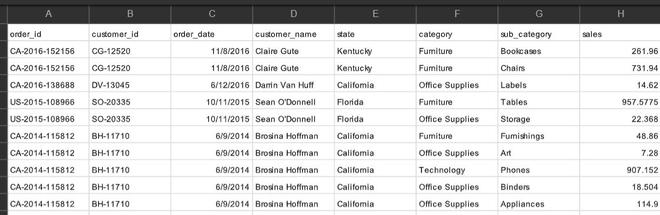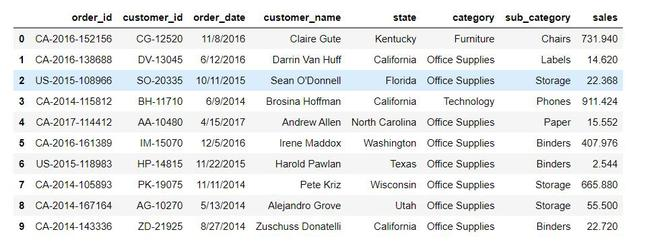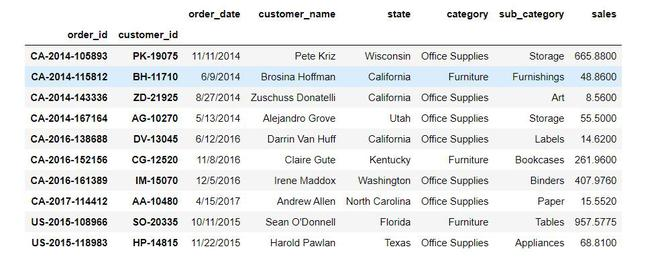Open in App
Not now

# Delete duplicates in a Pandas Dataframe based on two columns

• Last Updated : 11 Dec, 2020

A dataframe is a two-dimensional, size-mutable tabular data structure with labeled axes (rows and columns). It can contain duplicate entries and to delete them there are several ways.

Let us consider the following dataset.The dataframe contains duplicate values in column order_id and customer_id. Below are the methods to remove duplicate values from a dataframe based on two columns.

Method 1: using drop_duplicates()

Approach:

• We will drop duplicate columns based on two columns
• Let those columns be ‘order_id’ and ‘customer_id’
• Keep the latest entry only
• Reset the index of dataframe

Below is the python code for the above approach.

## Python3

 `# import pandas library``import` `pandas as pd`` ` `# load data``df1 ``=` `pd.read_csv(``"super.csv"``)`` ` `# drop rows which have same order_id``# and customer_id and keep latest entry``newdf ``=` `df1.drop_duplicates(``  ``subset ``=` `[``'order_id'``, ``'customer_id'``],``  ``keep ``=` `'last'``).reset_index(drop ``=` `True``)`` ` `# print latest dataframe``display(newdf)`

Output:Method 2: using groupby()

Approach:

• We will group rows based on two columns
• Let those columns be ‘order_id’ and ‘customer_id’
• Keep the first entry only

The python code for the above approach is given below.

## Python3

 `# import pandas library``import` `pandas as pd`` ` `# read data``df1 ``=` `pd.read_csv(``"super.csv"``)`` ` `# group data over columns 'order_id'``# and 'customer_id' and keep first entry only``newdf1 ``=` `df1.groupby([``'order_id'``, ``'customer_id'``]).first()`` ` `# print new dataframe``print``(newdf1)`

Output:My Personal Notes arrow_drop_up